# SAT II Math II : Median

## Example Questions

### Example Question #1 : Data Analysis And Statistics

Find the median of the following data set: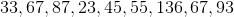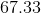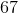Explanation:

Find the median of the following data set:First, put the numbers in ascending order: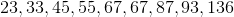Next, identify the median by identifying the middle term.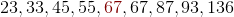So, our median is 67.

### Example Question #2 : Data Analysis And Statistics

Determine the median of the following numbers: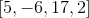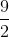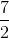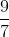Explanation:

Order the numbers from least to greatest.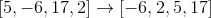The median of a even set of numbers is the average of the central two numbers.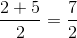The answer is:### Example Question #3 : Data Analysis And Statistics

Determine the median of the numbers: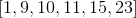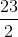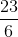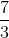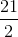Explanation:

The numbers provided are already in chronological order.

Since we have an even set of numbers, the median of the numbers is the average of the two central numbers.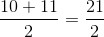Do not confuse the meaning of mean and median!

The answer is:### Example Question #1 : Median

Determine the median of the numbers: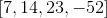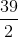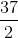Explanation:

Order the data set from least to greatest.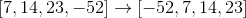The median is the average of the two central numbers for an even amount of numbers in the data set.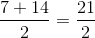The answer is:### Example Question #2 : Median

Determine the median of the following numbers: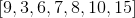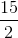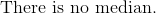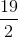Explanation:

Organize the numbers from least to greatest.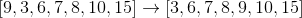The median of an odd set of numbers is the central number.

The answer is: